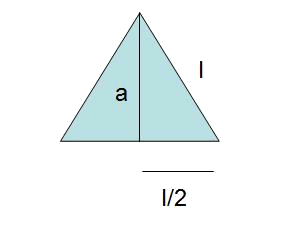# The regular polygon

The area of the regular polygon is $$A=\frac{Perimeter \cdot \ apothem}{2}$$$The perimeter is: $$P=n \cdot l$$$

with $$l$$ being the length of the radius and $$n$$ the number of sides.

Calculate the area of a hexagon of $$l=10 \ cm$$

• Using Pythagoras we find the apothem $$a$$, or the height of one of six equilateral triangles that form the hexagon.$$l^2= a^a+ \Big(\frac{l}{2}\Big)^2 \\ a^2= l^2 \cdot \Big(1-\frac{1}{4}\Big) \\ a= \frac{\sqrt{3}}{2} \cdot l$$$• The area of one of the triangles: $$A_{triangle}=\frac{l·a}{2} = l^2 \cdot \frac{\sqrt{3}}{4}= 25 \sqrt{3} \ cm^2$$$

• Finally, multiplying by $$6$$ we obtain the total area: $$A_{hexagon}=150\sqrt{3} \ cm^2$$\$

Note: To find areas of more complex irregular polygons, the philosophy will be the same: to break them down into triangles and to add the areas of the triangles.